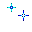#Bo Zhang's Homepage ..The universe is unfolding as it should..

## 2004-10-30

### FITS文件格式简介

FITS（Flexible Image Transport System）是天文学界常用的数据格式，它专门为在不同平台之间交换数据而设计。1988年的国际天文学联合会（IAU）大会指定IAU的FITS工作组全权负责此格式的修订。IAU规定，今后对FITS标准的修改不得破坏前后一致性，也就是所谓的“once FITS, always FITS”一说。

FITS文件由文件头和数据组成。在文件头中存储有对该文件的描述，如观测时间、观测对象、拍照温度、曝光时间等信息，同时也可以在文件头中注明观测时的视场、精度等，便于后期数据分析之用。文件头部分每行占80个字符，并以END结尾。按南京大学郑兴武教授所编《现代天体物理实验指导》一书中的说法，文件头共有36行，若未满则以空格补之。但我发现实际上某些FITS文件的头部要长于此值。每行的数据值一般为右对齐，“/”之后是注释内容。其具体表示格式是：

```SIMPLE  =                    T /
BITPIX  =                   16 /      Updated by AstroArt
NAXIS   =                    2 /
NAXIS1  =                  228
NAXIS2  =                  242
COMMENT
COMMENT
OBJECT  = 'M57 Nebula'
TELESCOP= 'Celestron C5'
EXPOSURE=                  120
APOLY0  =   4.94607111393E+000
APOLY1  =   5.77546902777E-001
APOLY2  =  -2.11265665729E-005
APOLY3  =  -4.11369637119E-006
APOLY4  =   2.70776116332E-003
APOLY5  =  -5.25005427724E-006
APOLY6  =   1.64728178524E-005
APOLY7  =  -1.27203899586E-003
CTYPE1  = 'RA---TAN'
CTYPE2  = 'DEC--TAN'
CRVAL1  =   2.83389000000E+002
CRVAL2  =   3.30910000000E+001
CRPIX1  =   1.07521861153E+002
CRPIX2  =   1.12170084259E+002
CD001001=  -1.21046310023E-003
CD001002=  -2.35697440267E-004
CD002001=  -3.00805952301E-004
CD002002=   9.43822939631E-004
CDELT1  =  -1.24727909385E-003
CDELT2  =   9.72807804616E-004
CROTA1  =   0.00000000000E+000
CROTA2  =   1.39885366995E+001
AVISUMIN=   1.56000000000E+002
AVISUMAX=   1.05200000000E+003
AVISUTYP=  -1.00000000000E+001
END                                                                              ```

http://fits.gsfc.nasa.gov/info_sources.html
 郑兴武, 现代天体物理实验指导